# The permanent conjecture

 Importance: Medium ✭✭
 Author(s): Kahn, Jeff
 Subject: Combinatorics » Matrices
 Keywords: invertible matrix permanent
 Recomm. for undergrads: no
 Prize: none
 Posted by: mdevos on: March 8th, 2007
Conjecture   If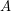is an invertiblematrix, then there is ansubmatrix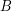ofso thatis nonzero.

If true, this conjecture would imply the nowhere-zero point in a linear mapping conjecture via the Alon-Tarsi polynomial technique. I believe Yang Yu was the first to suggest the following generalization of the permanent conjecture.

Conjecture  (Yu)   Ifare invertiblematrices over the same field, then there is ansubmatrix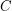of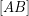so thatis nonzero.

This conjecture when restricted to the field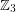is a consequence of the Alon-Tarsi basis conjecture. In addition to implying the above conjecture, the truth of this conjecture for matrices over the fieldwould imply that every 6-edge-connected graph has a nowhere-zero 3-flow, thus resolving The weak 3-flow conjecture.